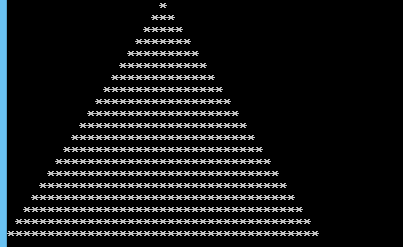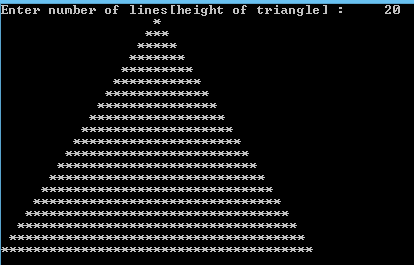## Saturday, 17 January 2015

### C Program to print a triangle filled with stars

This is a simple C Program which prints out a triangle with respect to the input given , the obtained triangle is  filled with stars.Triangle pattern

PROGRAM :
```#include <stdio.h>
#include <stdlib.h>

int main()
{
int i, j, n,k;
printf("Enter number of lines[height of triangle] : \t");
scanf("%d",&n);
for(i=1; i<=n; i++)
{
for(j=i; j<n; j++)
{
printf(" ");
}
for(k=1; k<i*2; k++)
{
printf("*");
}
printf("\n");
}
return 0;
}
```

OUTPUT :C Program to print a triangle